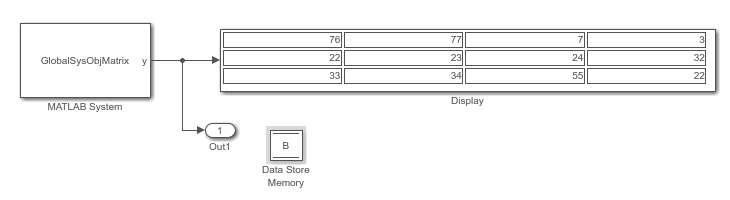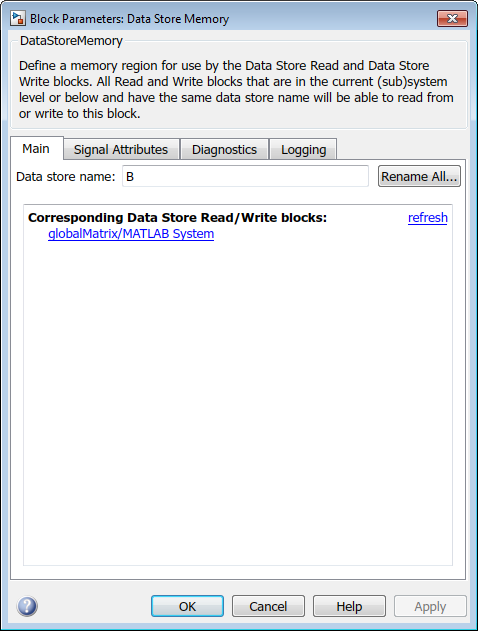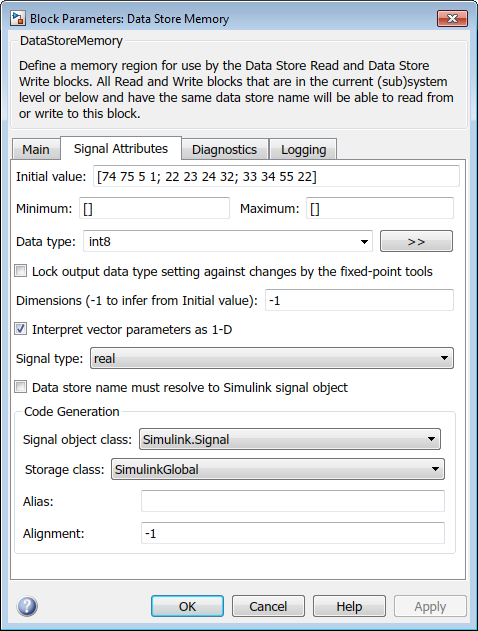## 在 System object 中使用全局变量

### Simulink 中的 System object 全局变量

MATLAB System 模块设置和使用全局变量的方式与对 MATLAB Function 模块的方式相同（请参阅数据存储 (Simulink)全局共享数据 (Simulink)）。与 MATLAB Function 模块一样，您也必须使用与 Data Store Memory 模块匹配的变量名称，才能在 Simulink 中使用全局变量。

```classdef GlobalSysObjMatrix < matlab.System methods (Access = protected) function y = stepImpl(obj) global B; B(1,:) = B(1,:)+1; y = B; end % Include getGlobalNamesImpl only if the class file is P-coded. function globalNames = getGlobalNamesImpl(~) globalNames = {"B"}; end end end ```